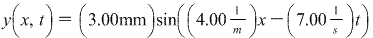# Problem: Use the wave equation to find the speed of a wave given by:

###### FREE Expert Solution

General wave solution:

$\overline{){\mathbf{y}}{\mathbf{\left(}}{\mathbf{x}}{\mathbf{,}}{\mathbf{t}}{\mathbf{\right)}}{\mathbf{=}}{\mathbf{A}}{\mathbf{s}}{\mathbf{i}}{\mathbf{n}}{\mathbf{\left(}}{\mathbf{k}}{\mathbf{x}}{\mathbf{-}}{\mathbf{\omega }}{\mathbf{t}}{\mathbf{\right)}}}$

Wave constant:

$\overline{){\mathbf{k}}{\mathbf{=}}\frac{\mathbf{2}\mathbf{\pi }}{\mathbf{\lambda }}}$

Angular frequency:

$\overline{){\mathbf{\omega }}{\mathbf{=}}{\mathbf{2}}{\mathbf{\pi }}{\mathbf{f}}}$

Wave velocity:

$\overline{){\mathbit{v}}{\mathbf{=}}{\mathbf{f}}{\mathbf{\lambda }}}$

Comparing the wave equation given to the general wave equation:

k = 4.00m-1

84% (233 ratings)###### Problem Details

Use the wave equation to find the speed of a wave given by: